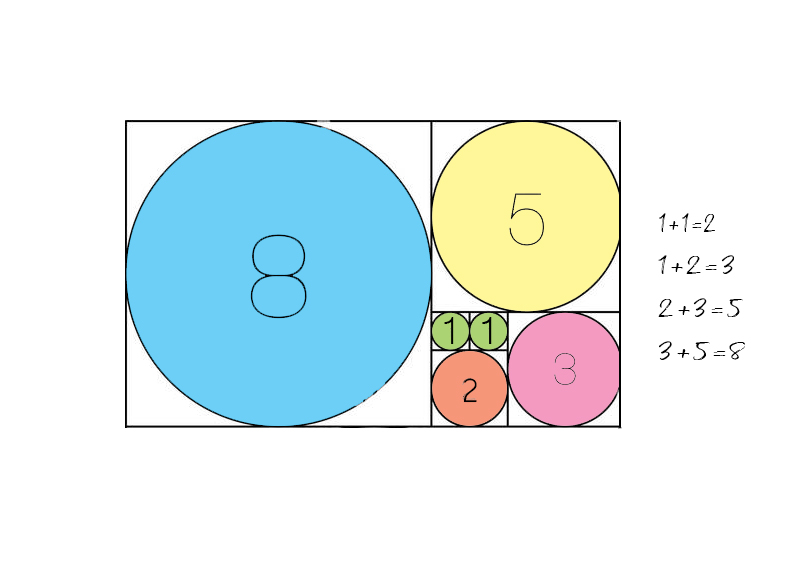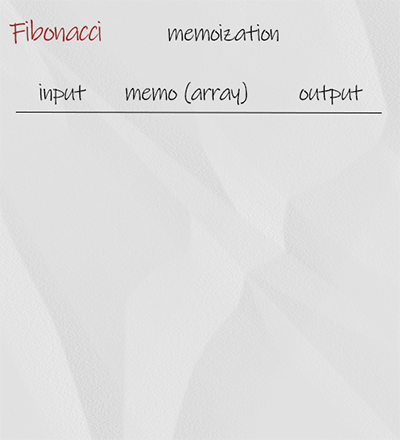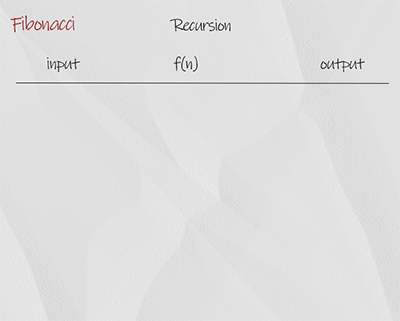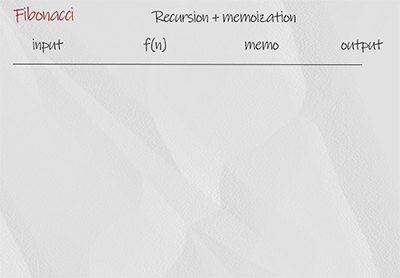Fibonacci numbers are a sequence of numbers, in which each number is the sum of the two preceding ones. F(0) = 0, F(1) =1, and F(n) = F(n-1) + F(n-2). The first few numbers are: 0, 1, 1, 2, 3, 5, 8, 13, 21… Given a number n , you can find n-th Fibonacci number using the following 4 implementations.### Iteration

This is the most efficient implementation. We use for loop starting from 2. In each iteration, variable sum in the sum of previous two number a and b. When finish, return sum.

## Doodle### Dynamic programming

The basic idea is the same as iteration. In additional, we use an array to save all the result. At the end we return the value with the index of the input number from the array.

## Doodle### Recursion

The recursion is a simple implementation of the concept, in which each number is the sum of the previous of two number. The base condition is when input is 0 or 1, it return the number itself. It has overlapping. For example, when the input is 4, it calls f(2) twice. The time complexity is 2^n, which is not efficient.

## Doodle### Recursion + memoization

This method is to overcome the overlapping in recursion using memoization. The solution uses an array to store the partial result. For example, if the result of f(2) is saved, next time the function finds the result in array and returns it. This technique is also called dynamic programming.

## Doodle